Author: Oscar Cronquist Article last updated on May 04, 2022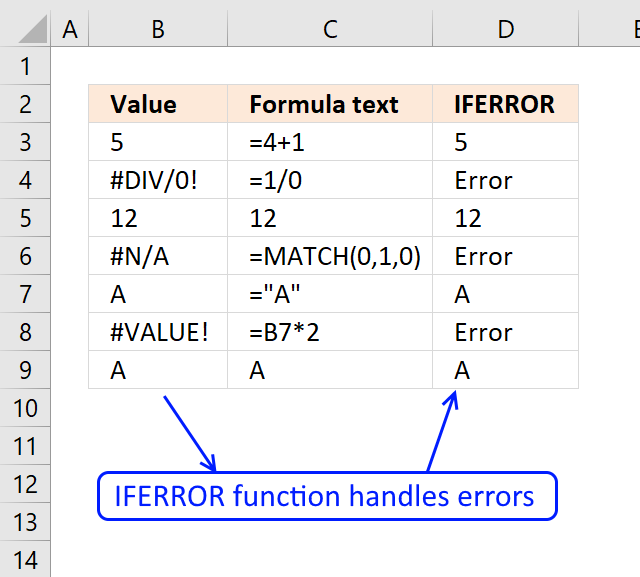The IFERROR function lets you catch most errors in Excel formulas. It was introduced in Excel 2007. In previous Excel versions, you could check for errors with the ISERROR function, it would return TRUE if the formula returned an error and FALSE if it was correct.

The IFERROR function makes it possible to create smaller formulas and still handle errors because the calculation is only needed once in the formula.

## 1. IFERROR Function Syntax

IFERROR(value, value_if_error)

## 2. IFERROR Function Arguments

 value A cell reference or a formula to check for errors. value_if_error The value to return if the value argument evaluates to an error. The following error types are evaluated: #N/A, #VALUE!, #REF!, #DIV/0!, #NUM!, #NAME?, or #NULL!.

If the value argument returns an error, the value_if_error argument is used. If the value argument does NOT return an error, the IFERROR function returns the value argument.

## 3. IFERROR Function ExampleFormula in cell D3:

=IFERROR(B3,"Error")

The formula returns "Error" if cell B3 evaluates an error. Column C shows what the corresponding cell contains in column B.

## 4. What errors doesn't the IFERROR function catch?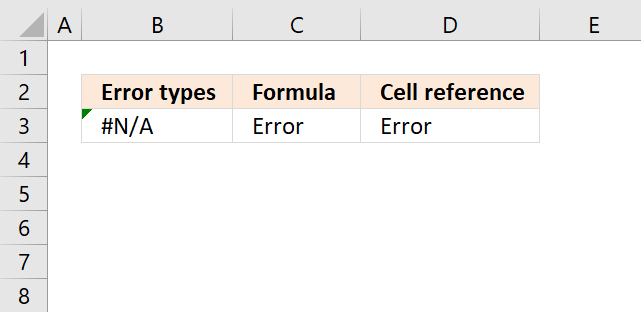The IFERROR function catches most errors except the #SPILL error and VBA errors in macros or UDFs.

Be careful using the IFERROR function, it will make it a lot harder to find errors in your worksheet that otherwise would be easy to spot.

## 5. How to catch the #N/A Error?

The image above shows a formula in cell B3 that returns a #N/A meaning "Value not available" error. The IFERROR function catches this error, however, if you want to detect exactly a #N/A error use the ISNA function or the IFNA function.

Formula in cell B2:

=MATCH(0,1,0)

This error is returned if a value is not found using one of these functions: MATCH, VLOOKUP, LOOKUP, HLOOKUP and XLOOKUP etc. The MATCH function looks for an exact match using 0 (zero) as the lookup value and 1 as the lookup array, it can't find the value and returns "#N/A".

The formula in cell C2 returns "error" if the value argument returns an error, in this case, it does.

Formula in cell C2:

=IFERROR(MATCH(0,1,0),"Error")

You can also reference a formula in another cell using the IFERROR function, this is demonstrated in cell D2.

Formula in cell D2:

=IFERROR(B2,"Error")

Cell B2 returns "#N/A" error and that makes the formula in cell D2 return "Error".

How to correct a #N/A error? The function you are using can't find the value you are looking for, try another lookup value or check that you reference the correct lookup array.

## 6. How to catch the #VALUE! error?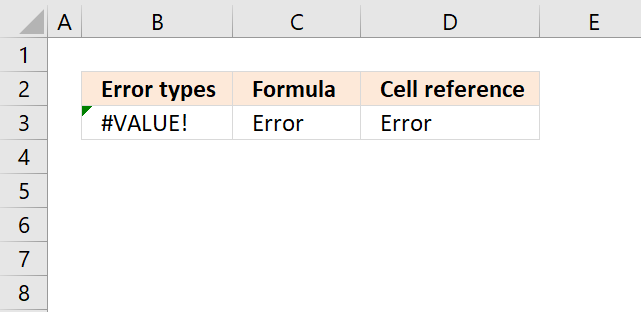The image above demonstrates a formula in cell B3 that returns the #VALUE! error. The formula tries to multiply a text string with a number, this mathematical operation is not valid.

Formula in cell B3:

=B2*2

How to correct the #VALUE! error? You have made a mistake when you built the function using arguments or one or more arguments are trying to do something that is not valid. For example. you perhaps are using text strings when the function only accepts numerical values.

Formula in cell C3:

=IFERROR(B2*2,"Error")

The IFERROR function catches the #VALUE! error as well, cell C3 returns "Error".

The Formula in cell D3 references cell B3 and returns "Error" because cell B3 evaluates to an error.

=IFERROR(B3,"Error")

## 7. How to catch the #REF Error?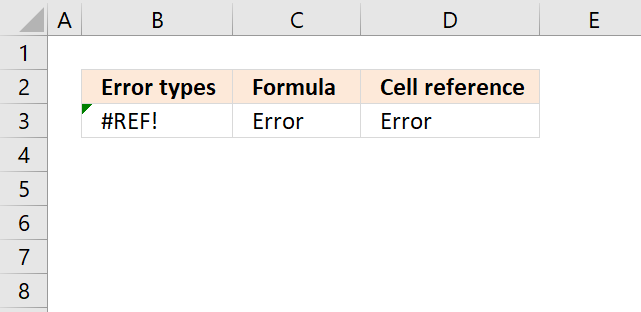The IFERROR function is able to catch #REF! errors, as well. The #REF! error is returned when you use an invalid cell reference.

Formula in cell B4:

=OFFSET(A1,-1,1)

The OFFSET function above tries to return a value from the row above cell A1 which is not possible. Excel returns a #REF! error.

Formula in cell C4:

=IFERROR(OFFSET(A1,-1,1),"Error")

How to correct the #REF! error? Often a cell reference is not valid, possibly been deleted or pointing to an incorrect location.

Formula in cell D4:

=IFERROR(B4,"Error")

The ISREF function checks if a value is a cell reference.

## 8. How to catch the #DIV/0 Error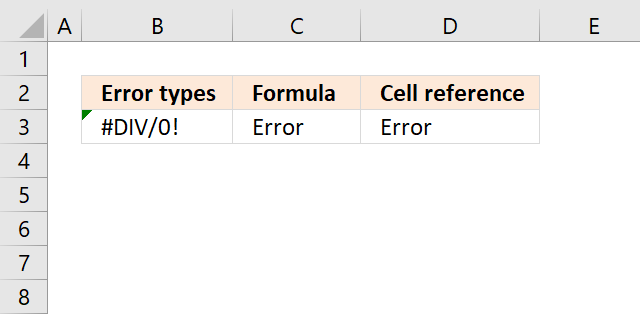The image above shows a formula in cell B3 that returns #DIV/0! error, this happens a formula tries to divide a number with 0 (zero).

Dividing a number with zero is not possible: Division by zero (wikipedia)

Formula in cell B5:

=1/0

The formula in cell B5 tries to divide 1 with 0 (zero), Excel returns the #DIV/0! error. How to correct a #DIV/0! error? Make sure that the divisor is not 0 (zero).

dividend/divisor = quotient

Formula in cell C5:

=IFERROR(1/0, "Error")

Formula in cell D5:

=IFERROR(B5, "Error")

## 9. How to catch the #NUM Error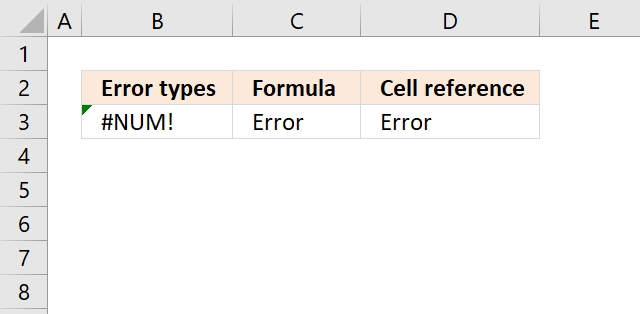The image above shows a formula in cell B3 that returns a #NUM! error. You get this result when you use an invalid number.

Formula in cell B7:

=NPER(7/12,-233,40000)

The formula in cell B7 returns #NUM! error, the second argument is a negative number which is not allowed. You can only positive numbers in this argument.

How to correct a #NUM! error? Check your function arguments carefully.

Formula in cell C7:

=IFERROR(NPER(7/12,-233,40000), "Error")

Formula in cell D7:

=IFERROR(B7, "Error")

## 10. How to catch the #NAME Error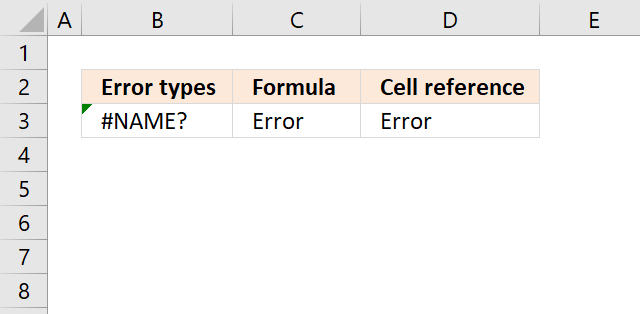The image above demonstrates a formula in cell B3 that returns #NAME! error.

Formula in cell B8:

=Text&1

The formula above tries to concatenate Text with 1 which is not possible.

How to correct a #NAME! error? There are many things that can be wrong here.

• Misspelled a named range.
• Misspelled a User Defined Function.
• Forgot to use double quotes before and after a text string.

Formula in cell C8:

=IFERROR(Text&1, "Error")

Formula in cell D8:

=IFERROR(B8, "Error")

## 11. How to catch the #NULL Error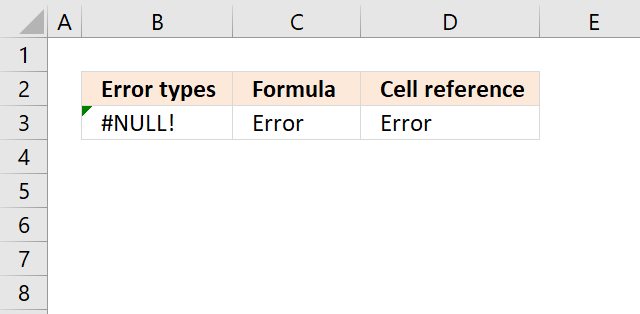You get the #NULL! error if you use an invalid cell reference in a formula or if you calculate the intersection of two cell ranges, however, they don't intersect.

Formula in cell B9:

=F3:G4 F6:G7

This formula calculates the intersection of two cell references using the space character. It returns a #NULL! error because they don't intersect meaning they don't cross or overlap.

How to correct a #NULL! error? Check your cell references and make sure that intersecting cell ranges cross each other.

Formula in cell C9:

=IFERROR(F3:G4 F6:G7, "Error")

Formula in cell D9:

=IFERROR(B9, "Error")

## 12. How can I find all errors in a worksheet?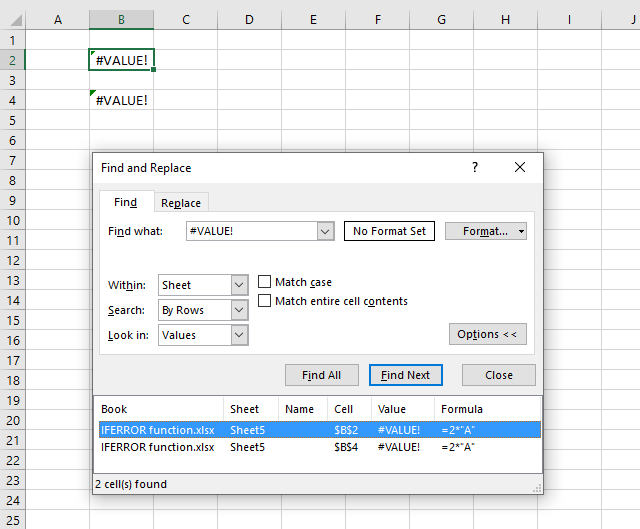Use the "Find & Replace" tool which is built-in to Excel, press short cut keys CTRL + F to launch it. A dialog box appears, enter the value you want to look for, in this example, #VALUE!

Press with left mouse button on "Options" button to see all settings. Change "Look in:" to Values, this is important or you won't find anything in your worksheet.

This method will, however, not catch errors inside the IFERROR function. As far as I know, you can't find those errors. I recommend that you use this method to locate all formulas using the IFERROR function and then, one by one, use the "Evaluate Formulas" tool to spot errors.

1. Select the cell containing the IFERROR function.
2. Go to tab "Formulas" on the ribbon.
3. Press with left mouse button on the "Evaluate Formulas" button.
4. Keep press with left mouse button oning the "Evaluate" button until you spot the error.
5. Press with left mouse button on "Close" button to dismiss the dialog box.
6. Repeat with the next cell.

## 13. IFERROR function simplifies formulas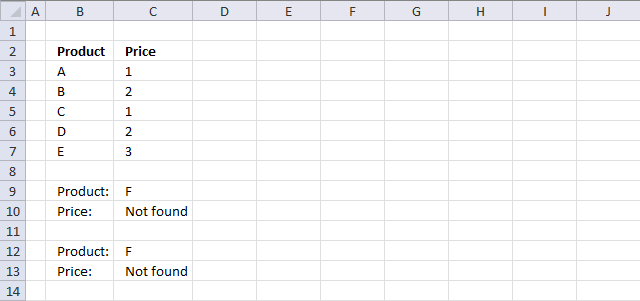Example 1

In previous Excel versions you could check for errors using the ISERROR function. This example shows both functions, the ISERROR function in cell C10 and IFERROR function in C12. The formula in cell C12 is much smaller.

Formula in cell C10:

Formula in cell C13:

Example 2

Here the price is missing for product A. The IFERROR function simplifies the formula in cell C13.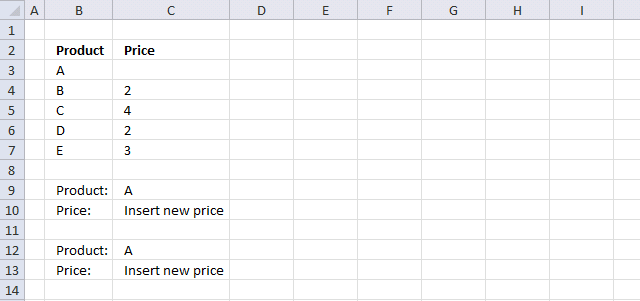Formula in cell C10:

=IF(INDEX(C3:C7,MATCH(C9,B3:B7,0))=0,"Insert new price",INDEX(C3:C7,MATCH(C9,B3:B7,0)))

Formula in cell C13:

=IFERROR(1/(1/(INDEX(C3:C7,MATCH(C12,B3:B7,0)))),"Insert new price")

### 13.1 Explaining the formula in cell C12

#### Step 1 - Find a value and return corresponding value

INDEX(C3:C7,MATCH(C12,B3:B7,0))

becomes

INDEX(C3:C7,MATCH("A",{"A";"B";"C";"D";"E"},0))

becomes

INDEX(C3:C7, 1)

becomes

INDEX({0;2;4;2;3}, 1)

#### Step 2 - Divide 1 with the returned value

=IFERROR(1/(1/(INDEX(C3:C7,MATCH(C12,B3:B7,0)))),"Insert new price")

becomes

=IFERROR(1/(1/0)),"Insert new price")

becomes

=IFERROR(1/#DIV/0),"Insert new price")

becomes

=IFERROR(#DIV/0,"Insert new price")

and returns "Insert new price" in cell C13.

### Get the Excel fileIFERROR-function.xlsx